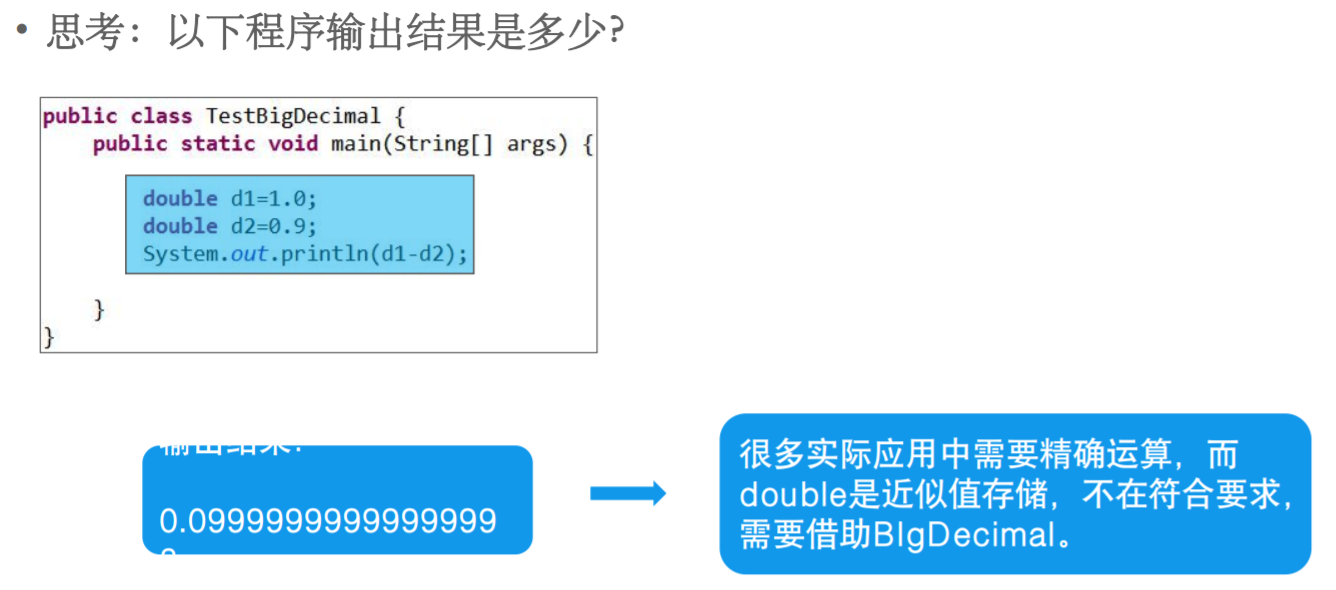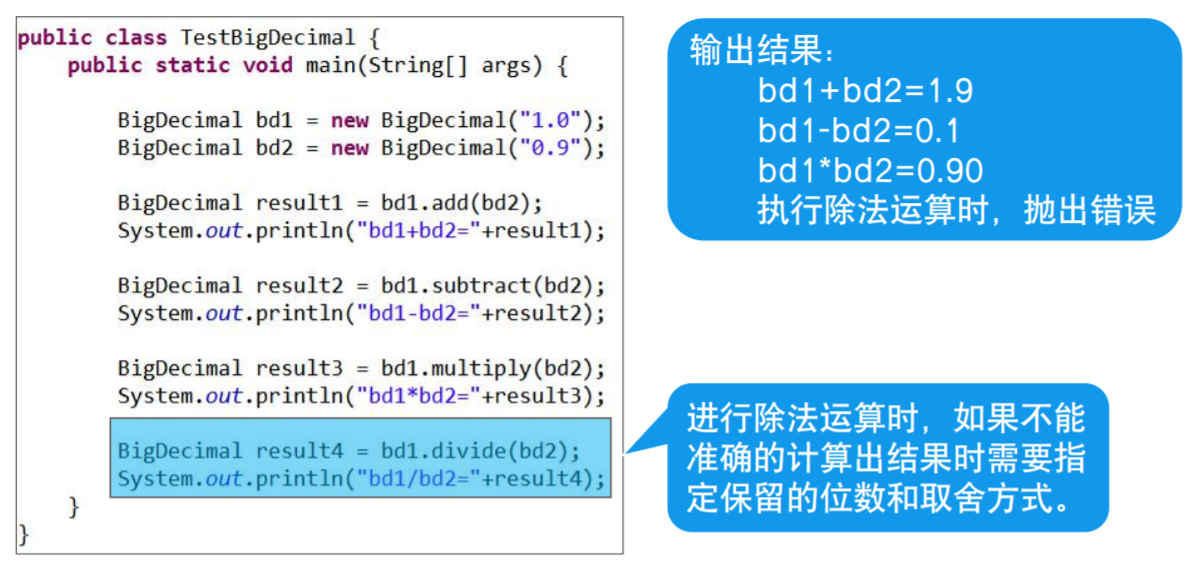• 关于Java怎么保留两位小数 一、打印时调用String类的format方法进行设置保留位数（方便简洁） 二、创建DecimalFormat对象来实现打印保留位数后的数字（比第二个多创建一个对象） 三、创建BigDecimal对象进行运算...
关于Java怎么保留两位小数
一、打印时调用String类的format方法进行设置保留位数（方便简洁）
二、创建DecimalFormat对象来实现打印保留位数后的数字（比第二个多创建一个对象）
三、创建BigDecimal对象进行运算后，设置保留位数。（此方法感觉不太实用）

一、String.format
double d1 = 6.6666;
double d2 = 8.88888;
double d3 = 3;
System.out.println(String.format("%.2f", d1));//设置保留两位小数
System.out.println(String.format("%.2f", d2));
System.out.println(String.format("%.2f", d3));
//注意：没有小数位或者小数位不够你设置的保留数时，自动补0


二、DecimalFormat
double d1 = 6.6666;
double d2 = 8.88888;
double d3 = 3;
DecimalFormat df = new DecimalFormat("#.00");//设置保留两位小数
System.out.println(df.format(d1));
System.out.println(df.format(d2));
System.out.println(df.format(d3));


三、BigDecimal位置：java.math包中
作用：精确计算浮点数
创建方式：BigDecimal bd = new BigDecimal(“1.0”);
方法：

BigDecimal subtract(BigDecimal bd)
BigDecimal multiply(BigDecimal bd)
BigDecimal divide(BigDecimal bd)

利用BigDecimal可以进行数值计算除法：BigDecimal(BigDecimal bd, int scal, RoundingMode mode)
参数scal：指定精确到小数点后几位
参数mode：

指定小数部分的取舍模式，通常采用四舍五入的模式
取值为BigDecimal.ROUND_HALF_UP


展开全文• 输入一个正整数repeat (0输入整数 x，计算并输出下列函数 f(x) 的值(保留2位小数，如果答案为3.50，则直接显示为3.5)。当 x 等于0时，f(x) = 0，当 x 不等于0时， f(x) = 1/x 。输入输出示例：括号内是说明输入3 ...
程序填空，不要改变与输入输出有关的语句。输入一个正整数repeat(0程序填空，不要改变与输入输出有关的语句。输入一个正整数repeat (0输入整数 x，计算并输出下列函数 f(x) 的值(保留2位小数，如果答案为3.50，则直接显示为3.5)。当 x 等于0时，f(x) = 0，当 x 不等于0时， f(x) = 1/x 。输入输出示例：括号内是说明输入3       (repeat=3)6       (x=6)10      (x=10)0       (x=0)输出f(6)=0.17f(10)=0.1f(0)=0.0import java.util.Scanner;public class Test30008{public static void main(String args[]){int repeat, ri,x;double y;Scanner in=new Scanner(System.in);repeat=in.nextInt();for(ri = 1; ri <= repeat; ri++){x=in.nextInt();/*---------*/System.out.println("f("+x+")="+y);}}}我写的import java.util.Scanner;public class Test30008{public static void main(String args[]){int repeat, ri,x;double y;Scanner in=new Scanner(System.in);repeat=in.nextInt();for(ri = 1; ri <= repeat; ri++){x=in.nextInt();/*---------*/if(x!=0)y=1/x;elsey=0;System.out.println("f("+x+")="+y);}}}应该怎么改啊？展开
展开全文• 今天，小编就来为大家讲一讲如何在Java保留小数点后两位。一、使用java.math.BigDecimal /*** 使用BigDecimal，保留小数点后两位*/public static String format1(double value) {BigDecimal bd = new BigDecimal(v...
小伙伴们在平时写程序的时，有没有需要在界面或者页面上保留小数点后两位的数据时候呢?今天，小编就来为大家讲一讲如何在Java中保留小数点后两位。一、使用java.math.BigDecimal /*** 使用BigDecimal，保留小数点后两位*/public static String format1(double value) {BigDecimal bd = new BigDecimal(value);bd = bd.setScale(2, RoundingMode.HALF_UP);return bd.toString();}二、使用java.text.DecimalFormat /*** 使用DecimalFormat,保留小数点后两位*/public static String format2(double value) {DecimalFormat df = new DecimalFormat("0.00");df.setRoundingMode(RoundingMode.HALF_UP);return df.format(value);}三、使用java.text.NumberFormat /*** 使用NumberFormat,保留小数点后两位*/public static String format3(double value) {NumberFormat nf = NumberFormat.getNumberInstance();nf.setMaximumFractionDigits(2);/** setMinimumFractionDigits设置成2** 如果不这么做，那么当value的值是100.00的时候返回100** 而不是100.00*/nf.setMinimumFractionDigits(2);nf.setRoundingMode(RoundingMode.HALF_UP);/** 如果想输出的格式用逗号隔开，可以设置成true*/nf.setGroupingUsed(false);return nf.format(value);}四、使用java.util.Formatter /*** 使用java.util.Formatter,保留小数点后两位*/public static String format4(double value) {/** %.2f % 表示 小数点前任意位数 2 表示两位小数 格式后的结果为 f 表示浮点型*/return new Formatter().format("%.2f", value).toString();}五、使用String.format /*** 使用String.format来实现。** 这个方法其实和format4是一样的*/public static String format5(double value) {return String.format("%.2f", value).toString();}完整代码及测试： import java.math.BigDecimal; import java.math.RoundingMode; importjava.text.DecimalFormat; import java.text.NumberFormat; importjava.util.Formatter;/**** @author wangmengjun**/public final class PrecisionTest {private PrecisionTest() {}/*** 使用BigDecimal，保留小数点后两位*/public static String format1(double value) {BigDecimal bd = new BigDecimal(value);bd = bd.setScale(2, RoundingMode.HALF_UP);return bd.toString();}/*** 使用DecimalFormat,保留小数点后两位*/public static String format2(double value) {DecimalFormat df = new DecimalFormat("0.00");df.setRoundingMode(RoundingMode.HALF_UP);return df.format(value);}/*** 使用NumberFormat,保留小数点后两位*/public static String format3(double value) {NumberFormat nf = NumberFormat.getNumberInstance();nf.setMaximumFractionDigits(2);/** setMinimumFractionDigits设置成2** 如果不这么做，那么当value的值是100.00的时候返回100** 而不是100.00*/nf.setMinimumFractionDigits(2);nf.setRoundingMode(RoundingMode.HALF_UP);/** 如果想输出的格式用逗号隔开，可以设置成true*/nf.setGroupingUsed(false);return nf.format(value);}/*** 使用java.util.Formatter,保留小数点后两位*/public static String format4(double value) {/** %.2f % 表示 小数点前任意位数 2 表示两位小数 格式后的结果为 f 表示浮点型*/return new Formatter().format("%.2f", value).toString();}/*** 使用String.format来实现。** 这个方法其实和format4是一样的*/public static String format5(double value) {return String.format("%.2f", value).toString();}} public class Main {public static void main(String[] args) {double[] testData = new double[] { 100.123D, 1234567.897D, 100.0052D,80.00D };for (double value : testData) {System.out.println(PrecisionTest.format1(value));System.out.println(PrecisionTest.format2(value));System.out.println(PrecisionTest.format3(value));System.out.println(PrecisionTest.format4(value));System.out.println(PrecisionTest.format5(value));}}}运行结果： 100.12100.12100.12100.12100.121234567.901234567.901234567.901234567.901234567.90100.01100.01100.01100.01100.0180.0080.0080.0080.0080.00以上就是今天关于Java如何保留小数点中后两位的介绍了，更多相关内容请继续关注本站吧。
展开全文• Java中，当两个整数相除时，由于小数点以后的数字会被截断，运算结果将为整数，此时若希望得到运算结果为浮点数，必须将两整数其一或是两者都强制转换为浮点数，也就是Java输出怎么保留两位小数?接下来郑州达内Java...
Java中，当两个整数相除时，由于小数点以后的数字会被截断，运算结果将为整数，此时若希望得到运算结果为浮点数，必须将两整数其一或是两者都强制转换为浮点数，也就是Java输出怎么保留两位小数?接下来郑州达内Java培训老师给大家以实例说明：Java怎么取两位小数?题目：项目中有一个小需求，两个整数相除，结果需要保留两位小数，即1.00、0.50这种数据格式。以下做法不行，因为两整数相除，小数点以后的数字会被截断，让运算结果为整数类型。int x = 10;int y = 20;int a = x/y;System.out.println(a);// 输出0System.out.println(x/y);// 输出0System.out.println((float)(x/y));// 输出0.0此时需要运算结果为浮点数，则此时必须将两整数其一或是全部强制转换类型为浮点数。float b = (float)x/y;float c = x/(float)y;float d = (float)x/(float)y;System.out.println(b);// 输出0.5System.out.println(c);// 输出0.5System.out.println(d);// 输出0.5为了控制保留两位小数，需要进行格式化，DecimalFormat df = new DecimalFormat("0.00");String e = df.format(b); // 此处可以用上面b/c/d任意一种System.out.println(e);// 输出0.50String e = df.format(a);System.out.println(e);// 输出0.00，由于a是int类型值，格式化之前x/y已经为整数0，因此此处只增加两位小数0网上有一种写法，用了如下方法，他的含义是四舍五入，保留两位小数，但需要小数有值。double a = new BigDecimal((float)10/20).setScale(2, BigDecimal.ROUND_HALF_UP).doubleValue();System.out.println(a);// 输出0.5double b = new BigDecimal((float)1/3).setScale(2, BigDecimal.ROUND_HALF_UP).doubleValue();System.out.println(f);// 输出0.33另外，float是单精度类型，精度是8位有效数字，内存分配4个字节，占32位，取值范围是10的-38次方到10的38次方，有效小数位6-7位。对应double是双精度类型，精度是17位有效数字，取值范围是10的-308次方到10的308次方，double占用8个字节的存储空间。默认小数都用double来表示，所以如果要用float的话，则应该在其后加上f，例如这么声明：float z=1.3;会报错误Type mismatch: cannot convert from double to float需要改为：float z=1.3f;
展开全文• java保留两位小数4种方法Java中保留小数有四种方法，如下：public class Format {double f = 23065.57845;public void m1() {BigDecimal bg = new BigDecimal(f);double f1 = bg.setScale(2, BigDecimal.ROUND_HALF_...
• http://blog.csdn.net/huaishuming/article/details/17752365**********************************************************4种方法，都是四舍五入，例：import java....import java.text.DecimalFormat;import java....
• 摘自http://irobot.iteye.com/blog/285537一Long是长整型，怎么小数，是double吧java.text.DecimalFormat df=new java.text.DecimalFormat("#.##");double d=3.14159;System.out.println(df.format(d));二java....
• 应该每个学java的人都知道java保留两位小数，如下： java.text.DecimalFormat df =new java.text.DecimalFormat("#.00"); df.format(数字); 可是，我想问的是： 例如： [code="java"] /* * java保留...企业应用
• java怎么用float类型数据保留两位小数发布时间：2020-06-23 21:01:33来源：亿速云阅读：235作者：Leahjava中怎么用float类型数据保留两位小数？针对这个问题，今天小编总结了这篇文章，希望能帮助更多想解决这个...
• import java.math.BigDecimal;import java.math.RoundingMode;import java.text.DecimalFormat;import java.text.NumberFormat;public class DoubleTest {/*** 保留两位小数，四舍五入的一个老土的方法* @pa...
• 展开全部JAVA中如何对double或者float的浮点数进行精度计算e68a843231313335323631343130323136353331333337396236，在JAVA中提供了多种参数来实现精度的不同控制方式.../*** 与小数位精度(四舍五入等)相关的一些常...
• 相信大家对内存数据库的 概念并不陌生，之前园子里也有多大牛介绍过SQL内存数据库的创建方法，我曾仔细 拜读过，有了大致了解，不过仍有很多细节不清晰，比如： （1）内存数据库是把整个数据库放到内存中的吗？ ...
• 怎么使float保留两位小数或多位小数  2011-11-28 11:58:34| 分类： java | 标签：float保留两位小数 |举报|字号 订阅 怎么使float保留两位小数或多位小数 两种方法： import ...
• 一个double型数字12.3659963 我想要12.37 怎么做呢 方法1 double i = 12.3659963;... //小数点后两位前移，并四舍五入 double k = (double)j / 100.00; //还原小数点后两位 方法2 double i=12.import string class
• 从这个观点可将整数分为种,一种叫质数,一种叫合成数.(有人认为数目字 1 不该称为质数)著名的高斯「唯一分解定理」说,任何一个整数.可以写成一串质数相乘的积.(例1) ,, , , , ,这就是说,任何...
• 怎么使float保留两位小数或多位小数两种方法：importjava.math.*;……方法1：floatf=34.232323;BigDecimalb=newBigDecimal(f);floatf1=b.setScale(2,BigDecimal.ROUND_HALF_UP).floatValue();//b.setScale(2,...
• 2016-09-01 01:16连东英 客户经理方式一：四舍五入double f = 111231.5585;BigDecimal b = new BigDecimal(f);double f1 = b.setScale(2, BigDecimal.ROUND...保留两位小数----------------------------------------...
• 问题：数据库中的数据为1.23456---------对应的----------java属性为Bigdecimal类型，在页面中显示时只保留小数点后两位，应该怎么做呢。 答案1：一部人因为经常用Bigdecimal用+-*/的精准运算，就会用如以下的工具...
• 5.怎么使float保留两位小数或多位小数* 两种方法： import java.math.*; …… 方法1： float f = 34.232323; BigDecimal b = new BigDecimal(f); float f1 = b.setScale(2, BigDecimal.ROUND_HALF_UP).float...textview
• java中取两位小数 但不要四舍五入是怎么做的？ 乘100再强转成int，再除以100就... //保留两位小数但不四舍五入 也可以写一个方法，以便重复使用：/** * a为一个带有未知位小数的实数 * 对其取b位小数 */static do...
• 之前一直没注意过Java怎么四舍五入。。。。太菜了 public static void main(String[] args) { DecimalFormat decimalFormat = new DecimalFormat("#.00"); double d = 17; double e = 17.6789; System.out....
• 题目：怎么在四舍五入后保留两位小数，小伙伴们知道如何解决吗?下面听小编来说道说道。一般有这四种方法，例：importjava.math.BigDecimal;importjava.text.DecimalFormat;importjava.text.NumberFormat;...
• 那么得出的结果就是 -0.00，四舍五入之后还是保留了符号，如果只是私有项目倒没什么问题但是如果是公司通过接口形式给前端的数据，前端就会显得很奇怪。 那么怎么也得有个处理或者加个 String res = String....# java怎么保留两位小数java 订阅